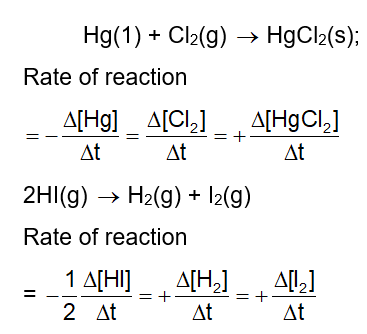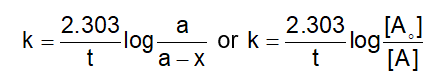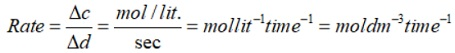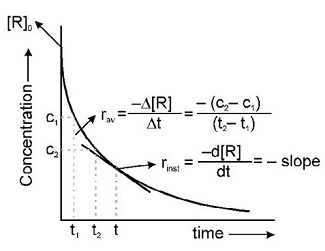# Chemistry formula for class 12 chapter- Chemical Kinetics

## Class 12 Chapter - Chemical Kinetic

• Rate of reaction: It is defined as the change in concentration of reactant (or product) in a particular time interval. Unit of rate of reaction is mol L–1 s–1.
• Average rate: The rate of reaction measured over a long time interval is called average rate of reaction. It is equal to △X/△t e.g• Instantaneous rate: It is the rate of reaction when the average rate is taken over a very small interval of time. It is equal to dx/dt.

Instantaneous rate = Average rate as △t approaches zero.

• Rate law or rate equation: It is the expression which relates the rate of reaction with concentration of the reactant. The constant of proportionality ‘K’ is known ass rate constant.
• Rate constant: When concentration of both reactant are unity, then the rate of reaction is known as rate constant. It is also called specific reaction rate.
• Molecularity: Total number of atoms, ions or molecules of the reactants involved in the reaction is termed as its molecularity. It is always in whole number. It is never more than three. It cannot be zero.

Example :

Unimolecular reaction

NH4NO2 →N2 + 2H2O

Bimolecular reaction

2HI(g) →H2(g) + I2(g)

Trimolecular reaction.

2NO(g) + O2(g) →2NO2(g)

• Order of a reaction: The sum of the exponents (power) of the concentration of reactants in the rate law is termed as order of the reaction. It can be in fraction. It can be zero also.
• Zero order reaction: The rate of reaction does not change with the concentration of reaction does not change with the concentration of the reactants, i.e., rate = k[A]°, where ‘k’ is rate constant Unit of rate constant is mol L–1 s–1.
• First order reaction: The reaction which the rate of reaction is directly proportional to the concentration of reacting substance. Rate constant of first order reaction isWhere ‘a’ is initial concentration, (a – x) is the conc. of reactants after time ‘t’. The unit of ‘k’ is s–1 or min–1. A plot between in [A] vs t is a straight line with slope equal to –k. [A] is concentration of reactants after time t. The unit of rate constant is s–1.

### List of Formulas of Chemical Kinetics

1. Velocity of Chemical Reaction1. Types of Rate of Chemical Reaction

R→PChemistry formula sheet for chapter-Chemical Kinetics is prepared by expert of Physics Wallah and consist of all important formula use in Chemical Kinetic chapter. Find NCERT solutions prepared by experts of Physics Wallah. If any students need to take the online test to check their concepts or undertstanding then they can visit Quiz for Chemical Kinetics. We also have Chemistry Doubts section where the most common doubts are explained by our expert faculty.

Download free pdf sheet which consist of formulas and important points of Chemical Kinetic from the link given below .